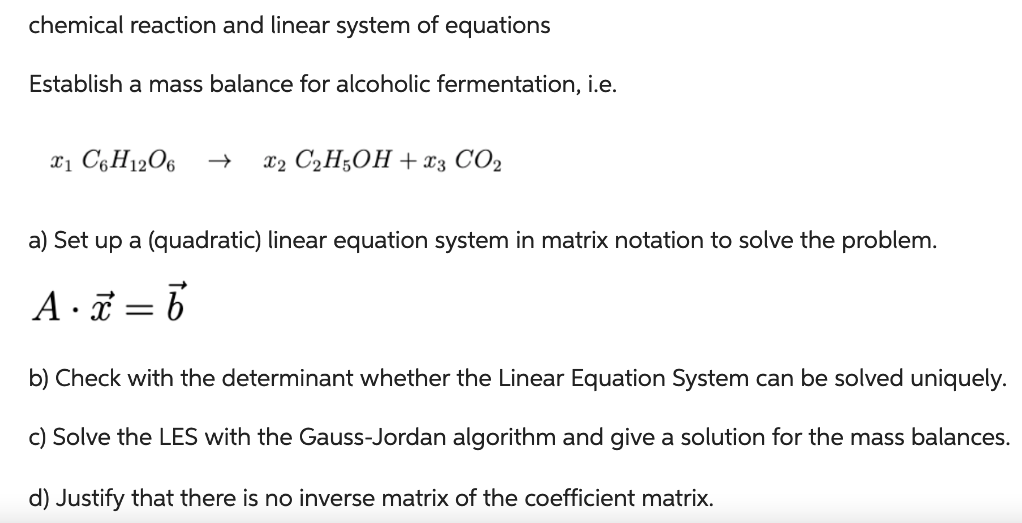Home / Expert Answers / Chemistry / chemical-reaction-and-linear-system-of-equations-nbsp-nbsp-if-you-could-hand-write-the-answ-pa837

# (Solved): chemical reaction and linear system of equations     If you could hand write the answ ...

chemical reaction and linear system of equationsIf you could hand write the answer I would appreciate it

chemical reaction and linear system of equations Establish a mass balance for alcoholic fermentation, i.e. $x_{1} \mathrm{C}_{6} \mathrm{H}_{12} \mathrm{O}_{6} \quad \rightarrow \quad x_{2} \mathrm{C}_{2} \mathrm{H}_{5} \mathrm{OH}+x_{3} \mathrm{CO}_{2}$ a) Set up a (quadratic) linear equation system in matrix notation to solve the problem. $A \cdot \vec{x}=\vec{b}$ b) Check with the determinant whether the Linear Equation System can be solved uniquely. c) Solve the LES with the Gauss-Jordan algorithm and give a solution for the mass balances. d) Justify that there is no inverse matrix of the coefficient matrix.

We have an Answer from Expert